# Helix

### From DT Online

##### Description

A Helix is the locus of a point travelling with constant linear velocity, axially along the outside of a cylinder as the cylinder rotates with constant angular velocity. The axial distance travelled by the point for each revolution of the cylinder is known as the pitch.

A helix is the curve used to produce a screw and is common in screwthreads and coil springs.

The elevation of this locus is a Sine Curve, which is also the curve used on a Displacement Diagram to generate Simple Harmonic Motion to a Cam Follower.

##### Constructions
 Drawing a Helix Start by drawing a circle to represent the diameter of the generating cylinder and divide it into equal sectors (say,12) Project horizontal lines along from each intersection between the radial dividing lines and the circle circumference. Make the length of these lines equal to the pitch of the helix to be drawn (or a mulitple of it if several ‘turns’ are needed). Start at 0 then plot Point 1 where the projection line from position 1 on the circle intersects the first vertical dividing line of the pitch as shown. Continue for all other points then sketch a fair curve through them to create the helix (or use French Curves or a flexible strip for example)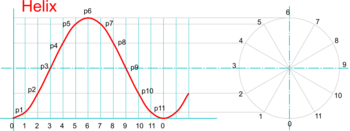Drawing Helical Screws and Springs Helical objects, such screwthreads and springs for example, are drawn in a similar way. Start by drawing two concentric circles: one equal to the outside diameter of the object and one equal to the inside diameter. Project horizontal lines from each circle as above and plot two helixes: one along the inner circle lines and one along those projected from the outer circle and sketch fair curves through these two to avoid confusion later. Choose a second starting point, furher along the pitch, and repeat to draw two more helixes as shown and sketch fair curves through them as before. Careful visualisation and selection of which parts of the helixes are in front and which are hidden will then enable a 3D screw-type form to be completed. ``` ```Note: To draw an actual thread or spring its cross-section must be drawn at the starting point 0 and this used to determine the inner (or ‘core’) diameter, the pitch, and the number of helixes required.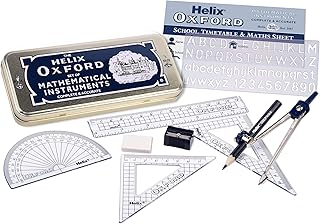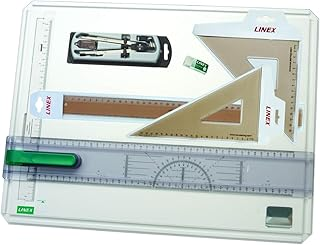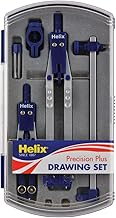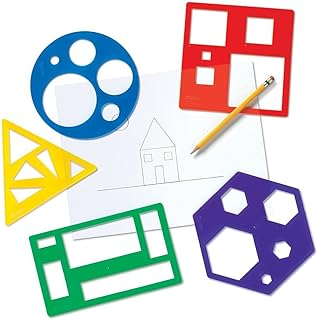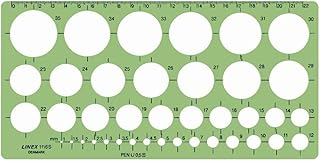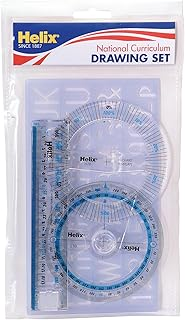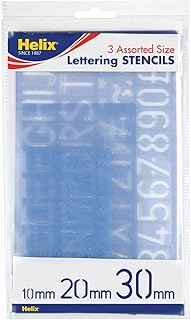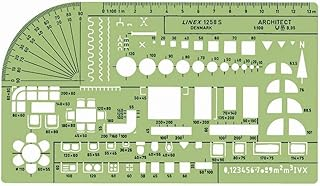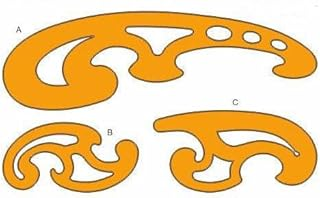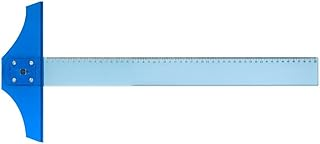Helix Oxford Maths SetLinex Student Saver BundleHelix Precision Plus Drawing SetPrimary Shape Template SetLinex Circle TemplateHelix National Curriculum Drawing SetHelix 3 Piece Stencil SetLinex Universal Architects TemplateFrench Curve Transparent TemplateT-Square